# Area of Triangle

Area of a triangle is the region enclosed by it, in a two-dimensional plane. As we know, a triangle is a closed shape that has three sides and three vertices. Thus, the area of a triangle is the total space occupied within the three sides of a triangle. The general formula to find the area of the triangle is given by half of the product of its base and height.

In general, the term “area” is defined as the region occupied inside the boundary of a flat object or figure. The measurement is done in square units with the standard unit being square meters (m2). For the computation of area, there are predefined formulas for squares, rectangles, circles, triangles, etc. In this article, we will learn the area of triangle formulas for different types of triangles, along with some example problems.

## What is the Area of a Triangle?

The area of a triangle is defined as the total region that is enclosed by the three sides of any particular triangle. Basically, it is equal to half of the base times height, i.e. A = 1/2 × b × h. Hence, to find the area of a tri-sided polygon, we have to know the base (b) and height (h) of it. It is applicable to all types of triangles, whether it is scalene, isosceles or equilateral. To be noted, the base and height of the triangle are perpendicular to each other. The unit of area is measured in square units (m2, cm2).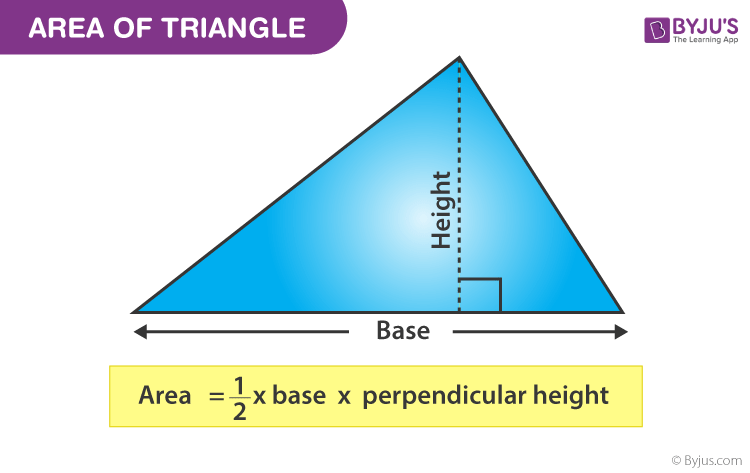Example: What is the area of a triangle with base b = 3 cm and height h = 4 cm?

Using the formula,

Area of a Triangle, A = 1/2 × b × h

= 1/2 × 4 (cm) × 3 (cm)

= 2 (cm) × 3 (cm)

= 6 cm2

Apart from the above formula, we have Heron’s formula to calculate the triangle’s area when we know the length of its three sides. Also, trigonometric functions are used to find the area when we know two sides and the angle formed between them in a triangle. We will calculate the area for all the conditions given here.

## Area of a Triangle Formula

The area of the triangle  is given by the formula mentioned below:

 Area of a Triangle = A = ½ (b × h) square units

where b and h are the base and height of the triangle, respectively.

Now, let’s see how to calculate the area of a triangle using the given formula. The area formulas for all the different types of triangles, like an area of an equilateral triangle, right-angled triangle, an isosceles triangle along with how to find the area of a triangle with 3 sides using Heron’s formula with examples are given below.

## Area of a Right Angled Triangle

A right-angled triangle, also called a right triangle has any one angle equal to 90°. Therefore, the height of the triangle will be the length of the perpendicular side.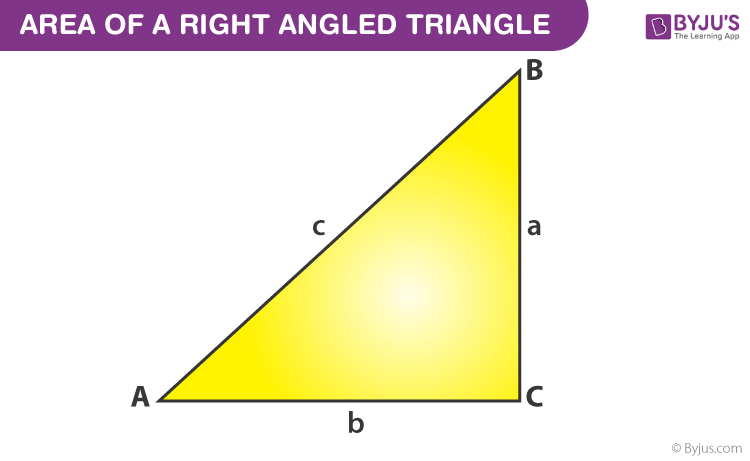Area of a Right Triangle = A = ½ × Base × Height (Perpendicular distance)

From the above figure,

Area of triangle ACB = 1/2 × a × b

## Area of an Equilateral Triangle

An equilateral triangle is a triangle where all the sides are equal. The perpendicular drawn from the vertex of the triangle to the base divides the base into two equal parts. To calculate the area of the equilateral triangle, we have to know the measurement of its sides.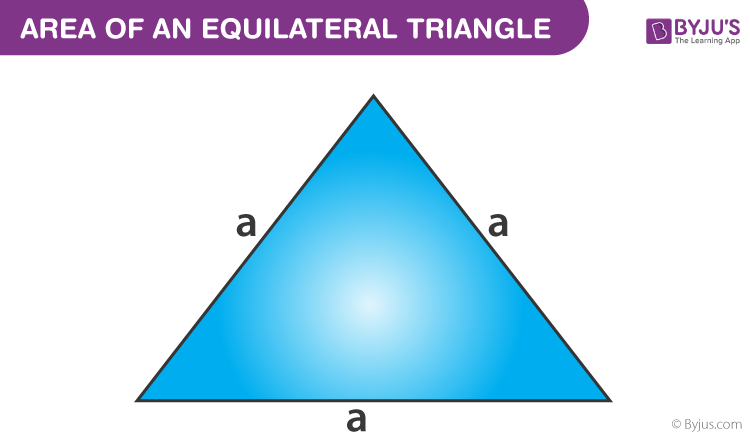• Area of an Equilateral Triangle = A = (√3)/4 × side2

## Area of an Isosceles Triangle

An isosceles triangle has two of its sides equal and also the angles opposite the equal sides are equal.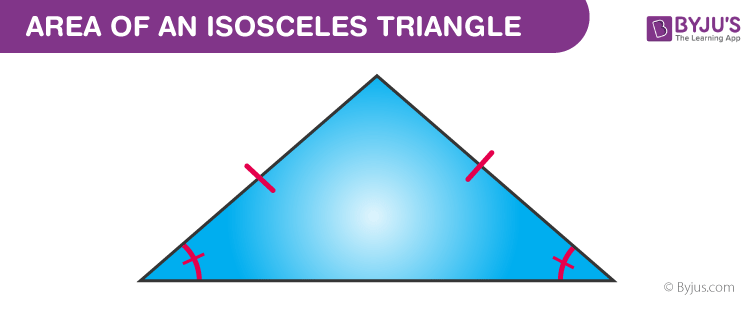$$\begin{array}{l}Area~ of~ an~ Isosceles~ Triangle = \frac{1}{4}b\sqrt{4a^{2}-b^{2}}\end{array}$$

## Perimeter of a Triangle

The perimeter of a triangle is the distance covered around the triangle and is calculated by adding all three sides of a triangle.

• The perimeter of a triangle = P = (a + b + c) units

where a, b and c are the sides of the triangle.

## Area of Triangle with Three Sides (Heron’s Formula)

The area of a triangle with 3 sides of different measures can be calculated using Heron’s formula. Heron’s formula includes two important steps. The first step is to find the semi perimeter of a triangle by adding all three sides of a triangle and dividing it by 2. The next step is to apply the semi-perimeter of triangle value in the main formula called “Heron’s Formula” to find the area of a triangle.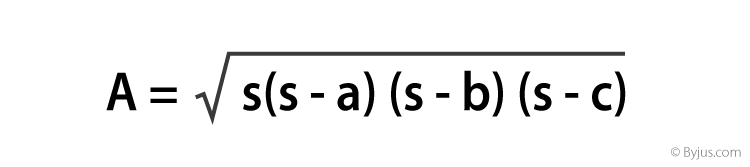where, s is semi-perimeter of the triangle = s = (a+b+c) / 2

We have seen that the area of special triangles could be obtained using the triangle formula. However, for a triangle with the sides being given, the calculation of height would not be simple. For the same reason, we rely on Heron’s Formula to calculate the area of the triangles with unequal lengths.

## Area of a Triangle Given Two Sides and the Included Angle  (SAS)

Now, the question comes, when we know the two sides of a triangle and an angle included between them, then how to find its area.

Let us take a triangle ABC, whose vertex angles are ∠A, ∠B, and ∠C, and sides are a,b and c, as shown in the figure below.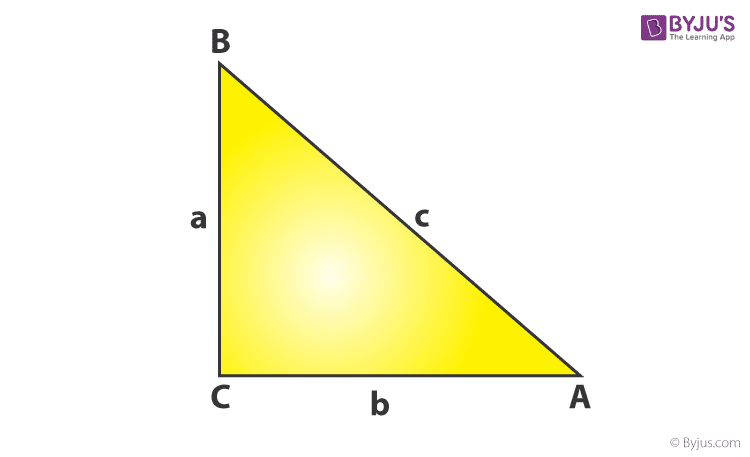Now, if any two sides and the angle between them are given, then the formulas to calculate the area of a triangle is given by:

Area (∆ABC) = ½ bc sin A

Area (∆ABC) = ½ ab sin C

Area (∆ABC) = ½ ca sin B

These formulas are very easy to remember and also to calculate.

For example, If, in ∆ABC,  A = 30° and b = 2, c = 4 in units. Then the area will be;

Area (∆ABC) = ½ bc sin A

= ½ (2) (4) sin 30

= 4 x ½   (since sin 30 = ½)

= 2 sq.unit.

## Area of a Triangle Solved Examples

Example 1:

Find the area of an acute triangle with a base of 13 inches and a height of 5 inches.

Solution:

A = (½)× b × h sq.units

⇒ A = (½) × (13 in) × (5 in)

⇒ A = (½) × (65 in2)

⇒ A = 32.5 in2

Example 2:

Find the area of a right-angled triangle with a base of 7 cm and a height of 8 cm.

Solution:

A = (½) × b × h sq.units

⇒ A = (½) × (7 cm) × (8 cm)

⇒ A = (½) × (56 cm2)

⇒ A = 28 cm2

Example 3:

Find the area of an obtuse-angled triangle with a base of 4 cm and a height 7 cm.

Solution:

A = (½) × b × h sq.units

⇒ A = (½) × (4 cm) × (7 cm)

⇒ A = (½) × (28 cm2)

⇒ A = 14 cm2

## Frequently Asked Questions on Area of a Triangle

Q1

### What is the area of a triangle?

The area of the triangle is the region enclosed by its perimeter or the three sides of the triangle.
Q2

### What is the area when two sides of a triangle and included angle are given?

The area will be equal to half times of the product of two given sides and sine of the included angle.
Q3

### How to find the area of a triangle given three sides?

When the values of the three sides of the triangle are given, then we can find the area of that triangle by using Heron’s Formula. Refer to the section ‘Area of a triangle by Heron’s formula‘ mentioned in this article to get a complete idea.

Q4

### How to find the area of a triangle using vectors?

Suppose vectors u and v are forming a triangle in space. Then, the area of this triangle is equal to half of the magnitude of the product of these two vectors, such that,

A = ½ |u × v|

Q5

### How to calculate the area of a triangle?

For a given triangle, where the base of the triangle is b and height is h, the area of the triangle can be calculated by the formula, such as;

A = ½ (b × h) Square Unit

Test your Knowledge on Area of Triangle

1. I cleared all my doubts because of this explaination. thank you byjus for thissimple explaination.

2. how to find area of a triangle if sum of squares of sides is given?

3. Can I take hypotenuse as a base in a right angled triangle?

4. How to find the area of equilateral triangle if the median is x cm?

5. VERY NICE QUESTION

6. If height is not given in a triangle how to find area

• you could use heron’s formula

7. awesome explanation byjus

8. This nice and really good app

9. how can u convert mm into cm millimetre into centimetre

• To convert mm into cm, divide the given value by 10.
1 mm = 1/10 cm = 0.1 cm

10. find the area of equilateral triangle whose side is 4 cm

• Area of equilateral triangle = √3/4a^2
If a = 4 cm, then,
area = √3/4 (4)^2
= 6.93 sq.cm. (Approx.)

11. If the height and base of a triangle are 24 cm and 46cm respectively, what is the area

• Area of the triangle = (1/2) * base * height
= (1/2) * 46 * 24
= 552 cm^2